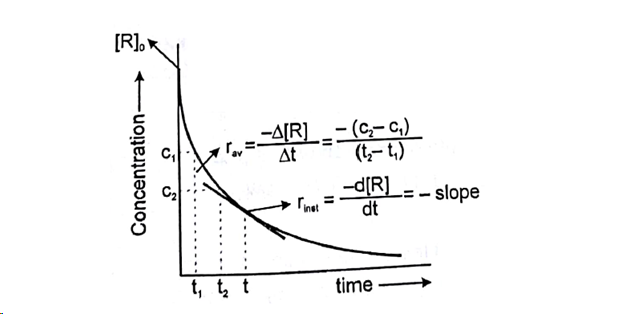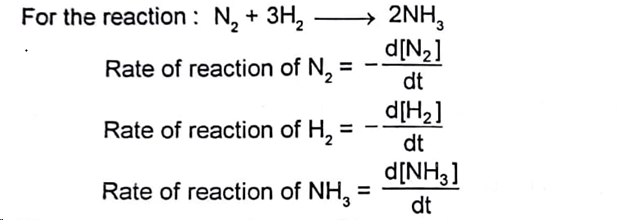Mona Thakur

teacher | Physics Chemistry

Description

Hi am Mona Teachers all the subjects from Class IX to Class XII as Follows. Class IX-X : Maths and Science. Class: XI-XII: Physics, Cheistry & Biology.

M.Sc

7+ Years

11-12

Current Organization

IIT Institute

Chemical reactions and factors affecting Rate

Chemical Reaction is a process that involves rearrangement of the molecular or ionic structure of a substance, as distinct from a change in physical form or a nuclear reaction.

Factors affecting rate are as follows:

1.      Concentration: We know from Law of mass action that Rate is proportional to concentration of reactions. So rate of reaction decreases with passage of time, since concentration of reactants decreases.

2.      Temperature: Effect of temperature on reaction rate was expressed in terms of temperature coefficient which was defined as the ratio of rate of reaction at two different temperature differing by 10% (usually these temperatures where taken as 25C and 30C)

3.      Nature of Reactants and Products

·         Physical state of reactants: Gaseous state > Liquid state > Solid state. Decreasing order of rate of reaction because collisions in homogeneous system are more effective than heterogeneous system.

·         Physical size of reactants: As we decrease the particle size rate of reaction increases since surface area increases.

·         Chemical nature of reactants: (a) If more bonds are to be broken, the rate of reaction will be slow. (b) Similarly bond strength is more, rate of reaction will be slow.

4.      Catalyst

·         Presence of positive catalyst lower down the activation energy hence increases the rate if reaction.

·         Presence of negative catalyst increases activation energy hence decreases the rate of reaction.

5.      PH of the solution·         This reaction takes place with appreciable rate in acidic medium, but does not takes place in basic medium.

6.      Dielectric constant of the medium: More is the dielectric constant of the medium greater will be the rate of iconic reactions.

8.      Pressure: Pressure is important factor of gaseous reaction.

9.      Electrical and magnetic field: Electric and magnetic fields are rate determining factors if a reaction involves polar species.

Note: First four factors generally affect rate of almost all reactions while other factors are specific to some reactions only.

Chemical Reaction: Rate/Velocity, Types of Rates, Relation between Reaction Rates

Rate/Velocity of chemical reaction

The rate of change of concentration with time of different chemical species taking part in a chemical reaction is known as rate of reaction of that species.Rate is always defined in such a manner so that it is always a positive quantity.

Types of Rates of chemical reaction

For a reaction R à P

Average rate = Total change in concentration / Total time Taken

Instantaneous Rate: Rate of reaction at a particular instant.

Instantaneous rate can be determined by drawing a tangent at time t on curve drawn for concentration versus time.

Initial Rate: Instantaneous rate ‘t = 0’ is called initial rate [slope of tangent at t = 0]Relation between reaction rates of different species involved is a reactionThese rates are not equal. Therefore, by convention the rate of a reaction is defined as

Note: Rate of reaction value is dependent on the stoichiometric coefficients used in the reaction while rate of any species will be fixed value under given conditions.

No Active Challenge

No Questions